﻿ 基于格子Boltzmann模型的Lee-Tarver爆轰波计算

# 基于格子Boltzmann模型的Lee-Tarver爆轰波计算The Computation of Lee-Tarver Detonation Based on Lattice Boltzmann Model

Abstract: In this paper, we present a high speed compressible lattice Boltzmann model coupled with Lee-Tarver reaction rate function for detonation. Two distribution functions are used to describe the density, momentum and energy of reactant and product in the lattice Boltzmann scheme, which gives consistent results with the Navier-Stokes equation in the continuum limit. Due to the separation of time scales in the chemical and thermodynamic process, the operator-splitting scheme is employed to solve Lee-Tarver reaction rate function. To indicate the validity of the model, we studied the collision between detonation and shock waves, the Richtmyer-Meshkov instability by detonation. The numerical examples show that the scheme can be used to compute the detonation phenomena.

1. 引言

2. 格子Boltzmann模型

2.1. 描述宏观流动的格子Boltzmann模型

$\left\{\begin{array}{l}{v}_{0}=0,\\ {v}_{ki}={v}_{k}\left[cos\left(i\text{π}/4\right),sin\left(i\text{π}/4\right)\right].\end{array}$ (1)

$\frac{\partial {f}_{ki}^{\sigma }}{\partial t}+{v}_{ki}\cdot \frac{\partial {f}_{ki}^{\sigma }}{\partial r}=-\frac{1}{{\tau }^{\sigma }}\left[{f}_{ki}^{\sigma }-{f}_{ki}^{\sigma ,eq}\right]$ . (2)

$\begin{array}{c}{f}_{ki}^{\sigma ,eq}={\rho }^{\sigma }{F}_{k}\left\{\left[1-\frac{{u}^{2}}{2T}+\frac{{u}^{4}}{8{T}^{2}}\right]+\frac{{v}_{ki\epsilon }{u}_{\epsilon }}{T}\left(1-\frac{{u}^{2}}{2T}\right)+\frac{{v}_{ki\epsilon }{v}_{ki\pi }{u}_{\epsilon }{u}_{\pi }}{2{T}^{2}}\left(1-\frac{{u}^{2}}{2T}\right)\\ \text{\hspace{0.17em}}\text{\hspace{0.17em}}+\frac{{v}_{ki\epsilon }{v}_{ki\pi }{v}_{ki\upsilon }{u}_{\epsilon }{u}_{\pi }{u}_{\upsilon }}{6{T}^{3}}+\frac{{v}_{ki\epsilon }{v}_{ki\pi }{v}_{ki\upsilon }{v}_{ki\xi }{u}_{\epsilon }{u}_{\pi }{u}_{\upsilon }{u}_{\xi }}{24{T}^{4}}\right\}.\end{array}$ (3)

$u=\frac{{\rho }^{r}{u}^{r}+{\rho }^{p}{u}^{p}}{{\rho }^{r}+{\rho }^{p}}$(4)

$T$ 表示局域平均温度，有

$T=\frac{{\rho }^{r}{T}^{r}+{\rho }^{p}{T}^{p}}{{\rho }^{r}+{\rho }^{p}}$(5)

$\underset{ki}{\sum }{f}_{ki}^{\sigma ,eq}={\rho }^{\sigma }$ , (6)

$\underset{ki}{\sum }{v}_{ki}{f}_{ki}^{\sigma ,eq}={\rho }^{\sigma }{u}^{\sigma }$ , (7)

$\underset{ki}{\sum }\frac{1}{2}{v}_{vi}^{2}{f}_{ki}^{\sigma ,eq}={e}_{therm}^{\sigma }+\frac{1}{2}{\rho }^{\sigma }{\left({u}^{\sigma }\right)}^{2}={\rho }^{\sigma }{T}^{\sigma }+\frac{1}{2}{\rho }^{\sigma }{\left({u}^{\sigma }\right)}^{2}={P}^{\sigma }+\frac{1}{2}{\rho }^{\sigma }{\left({u}^{\sigma }\right)}^{2}$ , (8)

$\underset{ki}{\sum }{v}_{ki\alpha }{v}_{ki\beta }{f}_{ki}^{\sigma ,eq}={e}_{therm}^{\sigma }{\delta }_{\alpha \beta }+{\rho }^{\sigma }{u}_{\alpha }^{\sigma }{u}_{\beta }^{\sigma }$ , (9)

$\underset{ki}{\sum }{v}_{ki\alpha }{v}_{ki\beta }{v}_{ki\gamma }{f}_{ki}^{\sigma ,eq}={e}_{therm}^{\sigma }\left({u}_{\gamma }^{\sigma }{\delta }_{\alpha \beta }+{u}_{\alpha }^{\sigma }{\delta }_{\beta \gamma }+{u}_{\beta }^{\sigma }{\delta }_{\gamma \alpha }\right)+{\rho }^{\sigma }{u}_{\alpha }^{\sigma }{u}_{\beta }^{\sigma }{u}_{\gamma }^{\sigma }$ ,(10)

$\underset{ki}{\sum }\frac{1}{2}{v}_{k}^{2}{v}_{ki\alpha }{f}_{ki}^{\sigma ,eq}=2{e}_{therm}^{\sigma }{u}_{\alpha }^{\sigma }+\frac{1}{2}{\rho }^{\sigma }{\left({u}^{\sigma }\right)}^{2}{u}_{\alpha }^{\sigma }$ , (11)

$\underset{ki}{\sum }\frac{1}{2}{v}_{k}^{2}{v}_{ki\alpha }{v}_{ki\beta }{f}_{ki}^{\sigma ,eq}=\left[2{T}^{\sigma }+\frac{1}{2}{\left({u}^{\sigma }\right)}^{2}\right]{e}_{therm}^{\sigma }{\delta }_{\alpha \beta }+\left[3{e}_{therm}^{\sigma }+\frac{1}{2}{\rho }^{\sigma }{\left({u}^{\sigma }\right)}^{2}\right]{u}_{\alpha }^{\sigma }{u}_{\beta }^{\sigma }$ . (12)

$\begin{array}{c}{f}_{kiI}^{\sigma ,new}={f}_{kiI}^{\sigma }-\frac{{c}_{ki\alpha }}{2}\left({f}_{kiI+1}^{\sigma }-{f}_{kiI-1}^{\sigma }\right)-\frac{\Delta t}{\tau }\left({f}_{kiI}^{\sigma }-{f}_{kiI}^{\sigma ,eq}\right)+\frac{{c}_{ki\alpha }^{2}}{2}\left({f}_{kiI+1}^{\sigma }-2{f}_{kiI}^{\sigma }+{f}_{kiI-1}^{\sigma }\right)\\ \text{\hspace{0.17em}}\text{\hspace{0.17em}}+\frac{{c}_{ki\alpha }\left(1-{c}_{ki\alpha }^{2}\right)}{12}\left({f}_{kiI+2}^{\sigma }-2{f}_{kiI+1}^{\sigma }+2{f}_{kiI-1}^{\sigma }-{f}_{kiI-2}^{\sigma }\right)+\frac{{\theta }_{\alpha I}^{\sigma }|{k}_{\alpha }^{\sigma }|\left(1-|{k}_{\alpha }^{\sigma }|\right)}{2}\left({f}_{kiI+1}^{\sigma }-2{f}_{kiI}^{\sigma }+{f}_{kiI-1}^{\sigma }\right).\end{array}$ (13)

2.2. 描述化学反应的Lee-Tarver反应率函数

$\frac{\text{d}\lambda }{\text{d}t}=\left\{\begin{array}{l}a\left(1-\lambda \right)+b\left(1-\lambda \right)\lambda ,\text{\hspace{0.17em}}\text{\hspace{0.17em}}T\ge {T}_{th},且0\le \lambda \le 1\\ 0,\text{\hspace{0.17em}}\text{\hspace{0.17em}}\text{\hspace{0.17em}}其它\end{array}$ (14)

$\frac{\partial \lambda }{\partial t}+u\nabla \lambda =0$ (15)

$\frac{{\lambda }_{I}^{n+1}-{\lambda }_{I}^{n}}{\Delta t}=-\left\{\begin{array}{l}\frac{u\left({\lambda }_{I}^{n}-{\lambda }_{I-1}^{n}\right)}{\Delta x},u\ge 0\\ \frac{u\left({\lambda }_{I+1}^{n}-{\lambda }_{I}^{n}\right)}{\Delta x},u<0\end{array}$ (16)

$\frac{\partial \lambda }{\partial t}=a\left(1-\lambda \right)+b\left(1-\lambda \right)\lambda$ , (17)

${\lambda }_{I}^{n+1}=\frac{{\text{e}}^{\left(a+b\right)\Delta t}+a\left({\lambda }_{I}^{n}-1\right)/\left(a+b{\lambda }_{I}^{n}\right)}{{\text{e}}^{\left(a+b\right)\Delta t}+b\left(1-{\lambda }_{I}^{n}\right)/\left(a+b{\lambda }_{I}^{n}\right)}$ . (18)

2.3. 化学反应与流动的耦合

$\stackrel{˙}{e}={\stackrel{˙}{e}}_{therm}+{\stackrel{˙}{e}}_{chem}$ , (19)

${\stackrel{˙}{e}}_{chem}=\stackrel{˙}{\lambda }\rho Q$ . (20)

${\rho }^{r,new}={\rho }^{r}-\stackrel{˙}{\lambda }\rho$ ,(21)

${\rho }^{p,new}={\rho }^{p}+\stackrel{˙}{\lambda }\rho$ .(22)

3. 数值例子

${\rho }_{0}\left(D-{u}_{0}\right)={\rho }_{1}\left(D-{u}_{1}\right)$ , (23)

${P}_{1}-{P}_{0}={\rho }_{0}\left(D-{u}_{0}\right)\left({u}_{1}-{u}_{0}\right)$ , (24)

${e}_{1}-{e}_{0}=0.5\left({P}_{1}+{P}_{0}\right)\left(1/{\rho }_{0}-1/{\rho }_{1}\right)+\lambda Q$ . (25)

${\left(\rho ,u,v,T,\lambda \right)}_{L}={\left(1.26,0.35,0,1.26,0\right)}_{L}$ ,

${\left(\rho ,u,v,T,\lambda \right)}_{M}={\left(1,0,0,1,0\right)}_{M}$ ,

${\left(\rho ,u,v,T,\lambda \right)}_{R}={\left(5.04,0,0,0.19,0\right)}_{R}$ .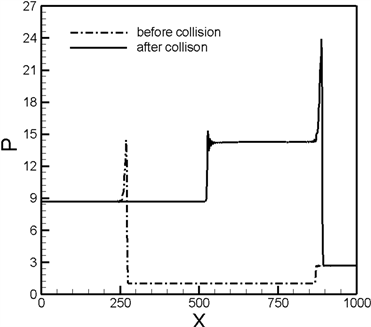(a)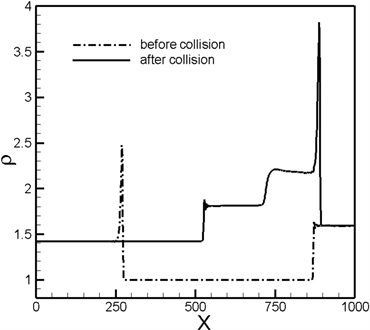(b)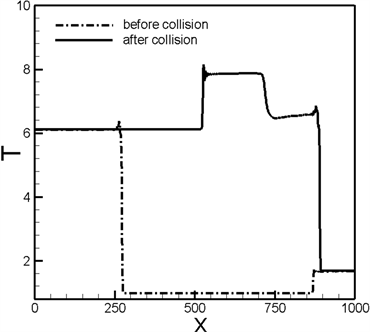(c)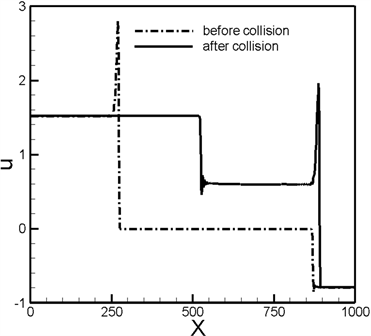(d)

Figure 1. Physical quantity profiles for before and after collision of detonation and shock. (a) Density $\rho$ , (b) pressure $P$ , (c) x-component of velocity $u$ , (d) mean temperature $T$Table 1. The Hugoniot relations before and after the transmitted detonation waveTable 2. The Hugoniot relations before and after the transmitted shock wave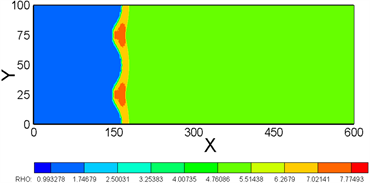(a)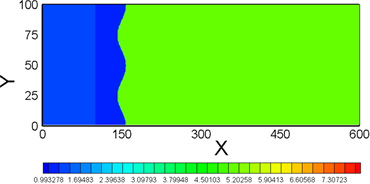(b)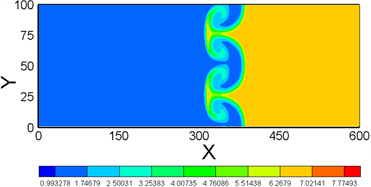(c)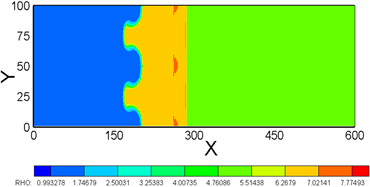(d)

Figure 2. Snapshots of density field for the Richtmyer-Meshkov instability in the case of detonation wave travels from heavy to light media. (a) t = 0, (b) t = 0.05, (c) t = 0.2, (d) t = 1.0

4. 结论

 Tryggvason, G., Bunner, B., Esmaeeli, A., Juric, D., et al. (2001) A Front-Tracking Method for the Computations of Multiphase Flow. Journal of Computational Physics, 169, 708-759.
https://doi.org/10.1006/jcph.2001.6726

 Glimm, J., Grove, J., Li, X. and Tan, D. (2006) Robust Computational Algorithms for Dynamic Interface Tracking in Three Dimensions. SIAM Journal on Scientific Computing, 21, 2240-2256.
https://doi.org/10.1137/S1064827598340500

 Mao, D. (2007) Towards Front-Tracking Based on Conservation in Two Space Dimensions II, Tracking Discontinuities in Capturing Fashion. Journal of Computational Physics, 226, 1550-1588.
https://doi.org/10.1016/j.jcp.2007.06.004

 Loubre, R., Maire, P., Shashkov, M., Breil, J. and Galera, S. (2010) ReALE: A Reconnection-Based Arbitrary- Lagrangian-Eulerian Method. Journal of Computational Physics, 229, 4724-4761.
https://doi.org/10.1016/j.jcp.2010.03.011

 Galera, S., Maire, P. and Breil, J. (2010) A Two-Dimensional Unstructured Cell-Centered Multi-materIal ALE Scheme Using VOF Interface Reconstruction. Journal of Computational Physics, 229, 5755-5787.
https://doi.org/10.1016/j.jcp.2010.04.019

 Osher, S. and Fedkiw, R.J. (2001) Level Set Methods: An Overview and Some Recent Results. Academic Press Professional, Inc., 169, 463-502.

 Sussman, M., Smereka, P. and Osher, S. (1994) A Level Set Approach for Computing Solutions to Incompressible Two-Phase Flow. Journal of Computational Physics, 114, 146-159.
https://doi.org/10.1006/jcph.1994.1155

 Scardovelli, R. and Zaleski, S. (2003) Direct Numerical Simulation of Free-Surface and Interfacial Flow. Annual Review of Fluid Mechanics, 31, 567-603.
https://doi.org/10.1146/annurev.fluid.31.1.567

 Succi, S., Bella, G. and Papetti, F. (1997) Lattice Kinetic Theory for Numerical Combustion. Plenum Press, 12, 395-408.

 Filippova, O. and Hanel, D. (2000) A Novel Lattice BGK Approach for Low Mach Number Combustion. Journal of Computational Physics, 158, 139-160.
https://doi.org/10.1006/jcph.1999.6405

 Filippova, O. and Hanel, D. (2000) A Novel Numerical Scheme for Reactive Flows at Low Mach Numbers. Computer Physics Communications, 129, 267-274.
https://doi.org/10.1016/S0010-4655(00)00113-2

 Yamamoto, K., He, X. and Doolen, G. (2002) Simulation of Combustion Field with Lattice Boltzmann Method. Journal of Statistical Physics, 107, 367-383.
https://doi.org/10.1023/A:1014583226083

 Yan, B., Xu, A.G., Zhang, G.C., Ying, Y.J. and Li, H. (2013) Lattice Boltzmann Model for Combustion and Detonation. Frontiers of Physics, 8, 94-110.
https://doi.org/10.1007/s11467-013-0286-z

 Lin, C.D., Xu, A.G., Zhang, G.C., Li, Y.J. and Succi, S. (2014) Polar-Coordinate Lattice Boltzmann Modeling of Compressible Flows. Physical Review E: Statistical Nonlinear & Soft Matter Physics, 89, 013307.
https://doi.org/10.1103/PhysRevE.89.013307

 Xu, A.G., Lin, C.D., Zhang, G.C. and Li, Y.J. (2015) Multiple-Relaxation-Time Lattice Boltzmann Kinetic Model for Combustion. Physical Review E: Statistical Nonlinear & Soft Matter Physics, 91, 043306.
https://doi.org/10.1103/PhysRevE.91.043306

 Watari, M. and Tsutahara, M. (2003) Two Dimensional Thermal Model of the Finite-Difference Lattice Boltzmann Method with High Spatial Isotropy. Physical Review E, 67, 036306
https://doi.org/10.1103/PhysRevE.67.036306

 Gan, Y.B., Xu, A.G., Zhang, G.C., Yu, X.J. and Li, Y.J. (2008) Two-Dimensional Lattice Boltzmann Model for Compressible Flows with High Mach Number. Physica A, 387, 1721-1732.
https://doi.org/10.1016/j.physa.2007.11.013

 Lee, E.L. and Tarver, C.M. (1980) Phenomenological Model of Shock Initiation in Heterogeneous Explosives. The Physics of Fluids, 23, 2362-2372.
https://doi.org/10.1063/1.862940

 Chen, F., Xu, A.G., Zhang, G.C., Gan, Y.B., Cheng, T. and Li, Y.J. (2009) Highly Efficient Lattice Boltzmann Model for Compressible Fluids: Two-Dimensional Case. Communications in Theoretical Physics, 52, 681-693.
https://doi.org/10.1088/0253-6102/52/4/25

 Chen, F., Xu, A.G., Zhang, G.C. and Li, Y.J. (2010) Three-Dimensional Lattice Boltzmann Model for High-Speed Compressible Flows. Communications in Theoretical Physics, 54, 1121-1128.
https://doi.org/10.1088/0253-6102/54/6/28

Top